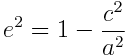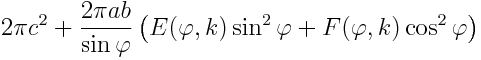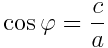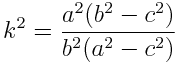# Approximating Earth as a sphere

Isaac Newton suggested in 1687 that the earth is not a perfectly round sphere but rather an ellipsoid, and he was right. But since our planet is roughly a sphere, it’s often useful to approximate it by a sphere. So if you’re going to do that, what radius do you use? More generally, what radius do you use when approximating any ellipsoid by a sphere?

This post will discuss the more general problem of finding the radius when approximating any ellipsoid by a sphere. We will give the answer for Earth in particular, and we’ll show how to carry out the calculations. Most of the calculations are easy, but some involve elliptic integrals and we show how to compute these in Python.

## Ellipsoids and spheroids

First of all, what is an ellipsoid? It is a surface whose (x, y, z) coordinates satisfyEarth is an oblate spheroid, which means a = b > c. Specifically, a = b = 6,378,137 meters, and c = 6,356,752 meters.

If you wanted to approximate an ellipsoid by a sphere, you could use

r = (a + b + c)/3.

Why? Because the knee-jerk reaction whenever you need to reduce a set of numbers to one number is to average them.

## Volume of an ellipsoid

We could do a little better, depending on what property of the ellipsoid we’d like to preserve in our approximation. For example, we might want to create a sphere with the same volume as the ellipsoid. In that case we’d use the geometric mean

r = (abc)1/3.

This is because the volume of an ellipsoid is 4πabc/3 and the volume of a sphere is 4πr3/3.

For the particular case of the earth, we’d use

(a2c)1/3 = 6371000.7

## Surface area of an ellipsoid

For some applications we might want a sphere with the same surface area as the ellipsoid rather than the same volume.

The surface area of an ellipsoid is considerably more complicated than the volume. For the special case of an oblate spheroid, like earth, the area is given bywhereThe surface area of a sphere is 4 πr2 and so the following code computes r.

```    from math import sqrt, atanh
e = sqrt(1 - (c/a)**2)
r = a*sqrt(1 + (1-e**2)*atanh(e)/e) / sqrt(2)
```

This gives r = 6371007.1 for the earth, about 6.4 meters more than the number we got matching volume rather than area.

For a general ellipsoid, the surface area is given bywhereandHere F is the “incomplete elliptic integral of the first kind” and E is the “incomplete elliptic integral of the second kind.” The names are historical artifacts, but the “elliptic” part of name comes from the fact that these functions were discovered in the context of arc lengths with ellipses, so it shouldn’t be too surprising to see them here.

## Computing ellipsoid surface area in Python

In SciPy, F(φ, k) is given by `ellipkinc` and E(φ, k) is given by `ellipeinc`. Both function names start with `ellip` because they are elliptic functions, and end in `inc` because they are “incomplete.” In the middle, `ellipeinc` has an “e” because it computes the mathematical function E(φ, k).

But why does `ellipkinc` have a “k” in the middle? The “complete” elliptic integral of the first kind is K(k) = F(π/2, k). The “k” in the function name is a reminder that we’re computing the incomplete version of the K function.

Here’s the code for computing the surface area of a general ellipsoid:

```    from math import sin, cos, acos, sqrt, pi
from scipy.special import ellipkinc, ellipeinc

def area(a, b, c):
phi = acos(c/a)
k = a*sqrt(b**2 - c**2)/(b*sqrt(a**2 - c**2))
E = ellipeinc(phi, k)
F = ellipkinc(phi, k)
elliptic = E*sin(phi)**2 + F*cos(phi)**2
return 2.0*pi*c**2 + 2*pi*a*b*elliptic/sin(phi)
```

The differences between the various approximation radii are small for Earth. See my next post on elliptical galaxies where the differences are much larger.

Related posts:

## 8 thoughts on “Approximating Earth as a sphere”

1. Troy McConaghy

Here’s a handy related fact about the Earth: the distance from a pole to the Equator is approximately 10,000 km.

2. Pseudonym

I can also imagine that the sphere which minimises the absolute error from celestial navigation would also be a useful one.

3. John Venier

Many globe knots are more naturally the surface of oblate or prolate spheroids than regular spheres. Of course, this has led many to tie some on oblate spheroid cores, and refer to the fact that the Earth is also an oblate spheroid, and suggest the knot is “Earth shaped”. But of course they are far more oblate than the Earth.

The fact that the Earth is an oblate spheroid is mentioned so often that I think many people think it looks like it got squashed. But the deviation of the Earth from a sphere is so slight it still looks just like a sphere from a distance. If someone handed you a ball in it’s shape, you would probably need calipers to tell it wasn’t a sphere.

One thing I have wondered about for a long time is if you had a billiard ball in the shape of the Earth, how straight would it roll on a pool table? Could you even tell from rolling it that it was not a sphere?

4. Your comment about a billiard ball reminds me of something that startled me as a boy. I read in a trivia book that if you shrunk the earth to the size of a billiard ball, you couldn’t feel mountains etc. Now that makes sense, but at the time it was surprising, possibly because of feeling globes with raised mountains greatly exaggerated from scale.

I don’t know, but I suspect the ellipticity of such a billiard ball might be noticeable. I don’t think you would feel it or see it, but you might observe that it rolls unusually.

5. A statistical measure might be to integrate the squared distances between the surfaces: || sphere(theta,phi) – ellipsoid(theta,phi) ||^2 where (theta,phi) are the angles in spherical coordinates. In other words, intersect the surfaces by a ray, calculate the squared distance between the surfaces in that direction, and integrate over all directions. [And maybe normalize it to get a mean integrated squared error (MISE)?]

6. Billiard balls are approximately 60 mm diameter, so the scaled difference in radius would be 30 (for a and b) vs 30*6356752/6378137 ~ 29.899 (for c). I’m not sure if 0.11 mm would be within the manufacturer’s tolerance to avoid wobbly balls, but I doubt I would notice with my poor billiard skills.

7. A quick google tells me that the tolerances for a snooker ball are 0.05mm in the diameter. So no, the earth would not be an acceptable billiard ball.